Author: Oscar Cronquist Article last updated on June 07, 2022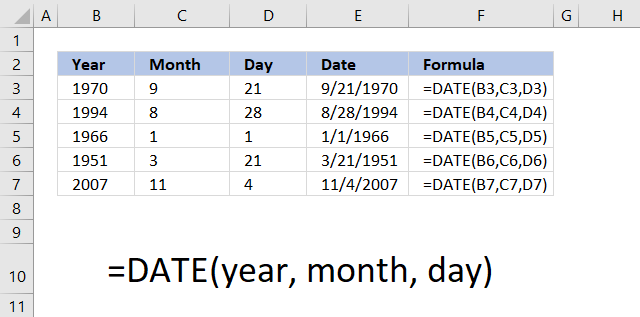The DATE function returns a number that acts as a date in the Excel environment. The image above shows you the DATE function in column E. It uses three arguments, the first argument is the year found in column B.

The second argument is the month, shown in column C. Note that you need to use a number for the month.

The third argument is the day of the month, displayed in column D. Make sure you enter the correct day for the month, keep in mind most months have 30 days or 31 days. February has 28 days except for leap years that have 29 days.

## 1. Excel function syntax

DATE(year, month, day)

## 2. Arguments

 year A number equal or higher than 1900. month A number between 1 (January) and 12 (December). You can use larger numbers as well. day A number between 1 and 31 based on the number of days in the month specified in argument month.

## 3. Add or subtract months and days

If you use a month number larger than 12, for example 13, DATE(2009,13,1) the DATE function returns 1/1/2010. Month number zero returns the previous month.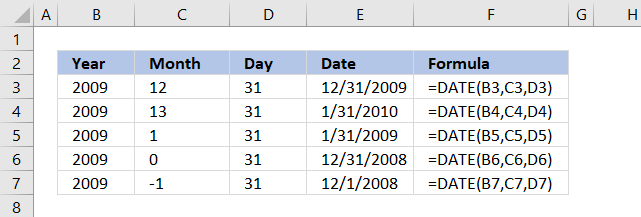The same thing happens with a day number that is larger than 31 or smaller than 1.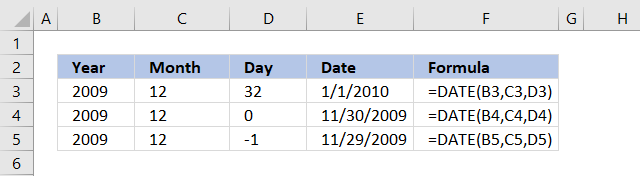You can use that to do dynamic date series or do date-based calculations.

## 4. Date function exampleThe image above demonstrates the date function in column E, it uses values from column B, C and D.

Formula in cell F3:

=DATE(B3, C3, D3)

## 5. What does the Date function do?The DATE function returns an Excel date that you can use in formulas, Excel can't calculate dates in formulas unless you reference a cell containing an Excel date or create an Excel date using the DATE function.

Hardcoding dates in a formula are not possible, use the DATE function to create an Excel date.

Why is that?
Excel uses numbers formatted as dates, 1 is 1/1/1900, and 1/1/2000 is 36526.

Prove it?
Sure, type 1 in a cell and press Enter. Select the cell and press CTRL + 1 to format the cell.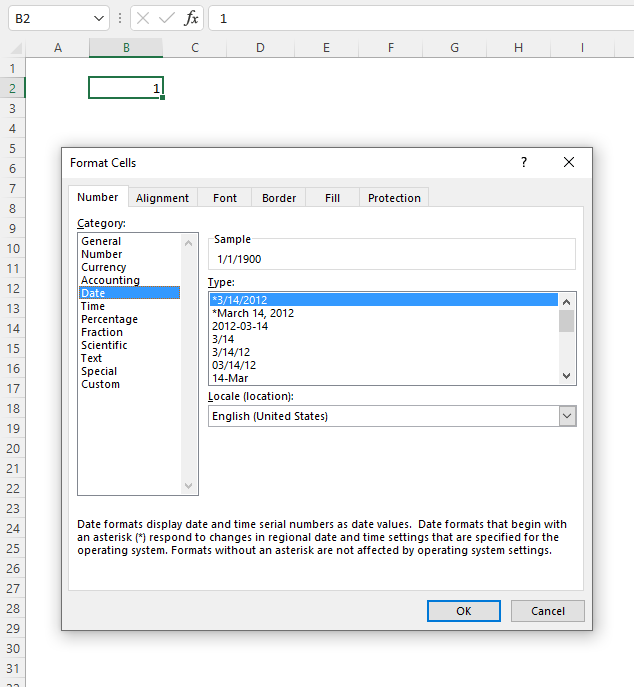A dialog box appears, select Date in Category: and press the "OK" button.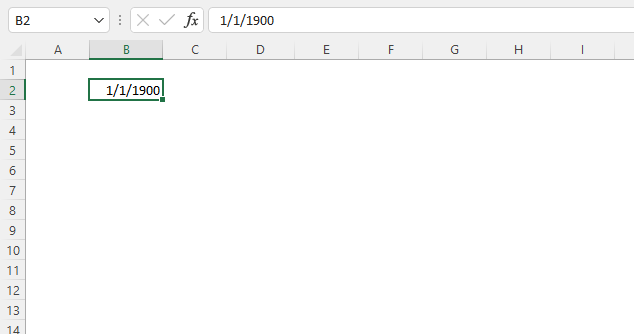Number 1 is now formatted as an Excel date, the cell shows 1/1/1900.

## 6. Date function format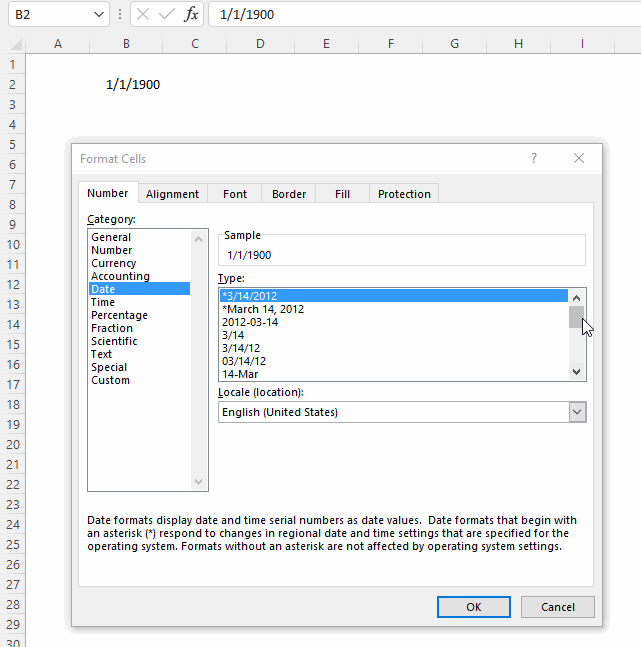The animated image above shows you the "Format Cells" dialog box, it allows you to choose from many different date formatting types. Here is how:

1. Select the cell.
2. Press CTRL + 1 to open the "Format Cells" dialog box.
3. Select Date in the Category:, see the image above.
4. Pick a formatting type.
5. Press with left mouse button on "OK" button to apply changes.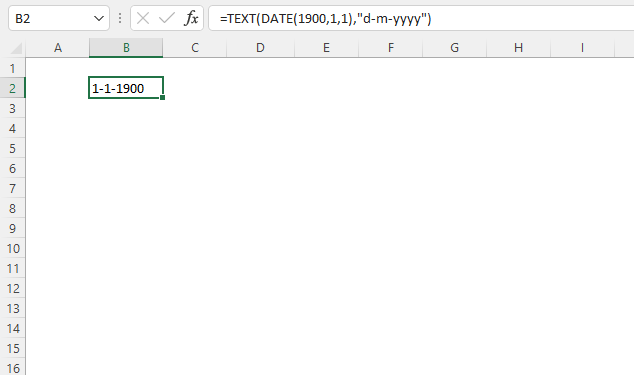You can also use the TEXT function to format dates.

Formula in cell B2:

=TEXT(DATE(1900, 1, 1), "d-m-yyyy")

### Explaining formula in cell B2

#### Step 1 - Create an Excel date

The DATE function calculates a serial number that represents a specific date based on three arguments. Year, month, and day.

DATE(year, month, day)

DATE(1900, 1, 1)

returns 1.

#### Step 2 - Return a number formatted as a date

The TEXT function formats a cell value based on given placeholder characters.

TEXT(value, format_text)

TEXT(DATE(1900, 1, 1), "d-m-yyyy")

becomes

TEXT(1, "d-m-yyyy")

and returns 1-1-1900.

## 7. Date function not working

1. Did you spell the DATE function correctly?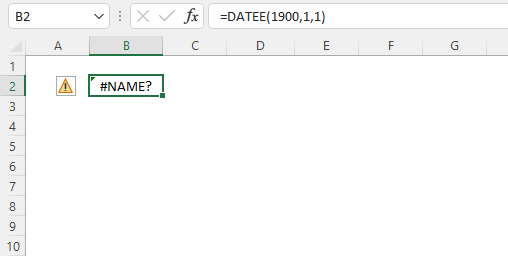Excel returns a #NAME? error if you didn't.
2. Check the arguments, they must be numerical. Text is not allowed.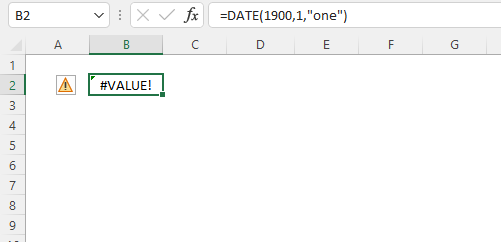Excel returns a #VALUE! error if any of the arguments contain a text value.
year - a number equal or larger than 0 (zero)
month - any number positive or negative
day - any number positive or negative
3. Did you use exactly three arguments?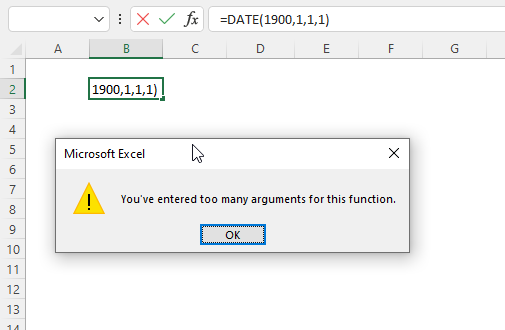Excel returns a dialog box: You've entered too many arguments for this function.
DATE(year, month, day)
4. Excel Date function returns the wrong date.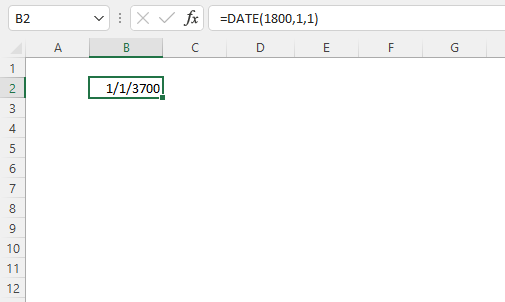If you try a year before 1900 Excel returns a date that you probably didn't expect. Here is how Excel came up with that date. 1900+1800 = 1/1/3700
5. The DATE function returns hashtags.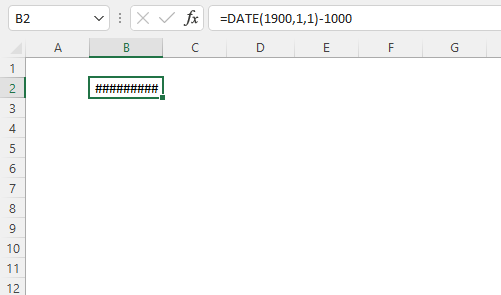The DATE function can't return dates before 1/1/1900. The formula in cell B2 above tries to calculate an Excel date before 1/1/1900.

## 8. How to make Excel order by date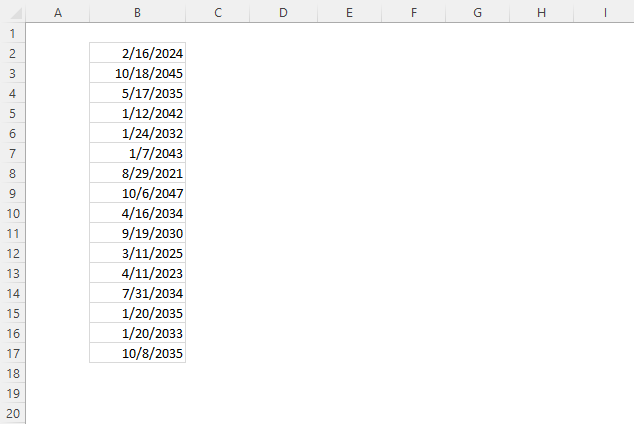The image above shows a list of dates in column B, here is how to sort the dates.

1. Press with right mouse button on any date in column B.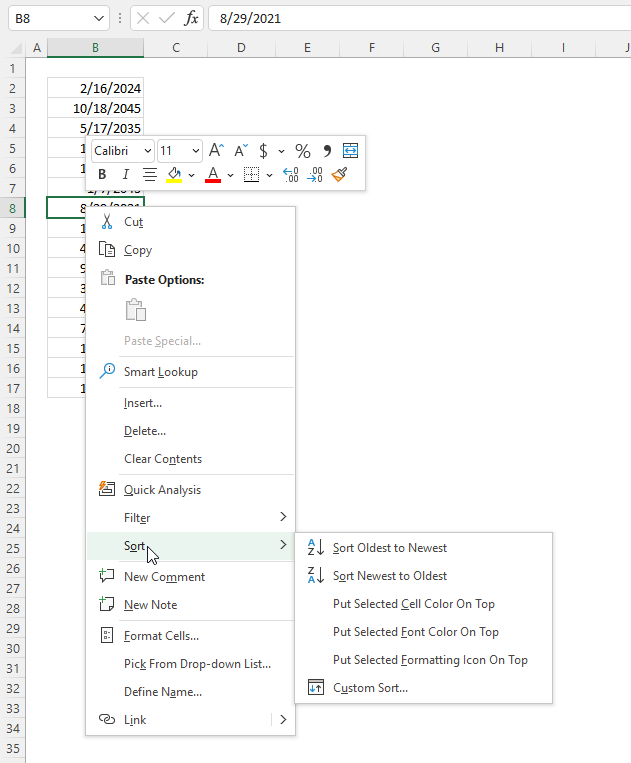2. Press "Sort". Another popup menu appears.
3. Press either "Sort Oldest to Newest" or "Sort Newest to Oldest".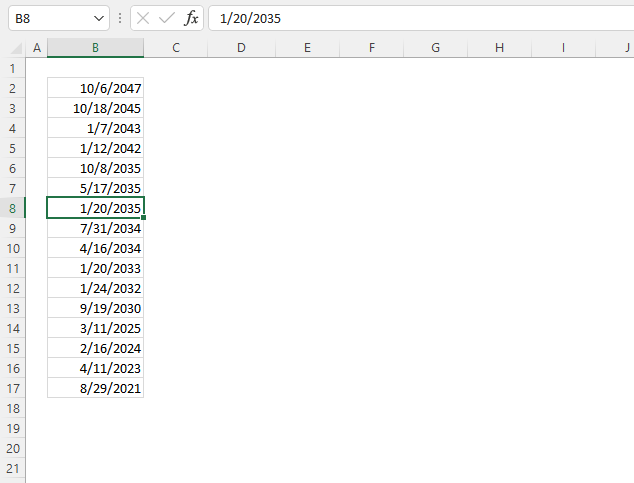Here is how to sort the dates using a formula.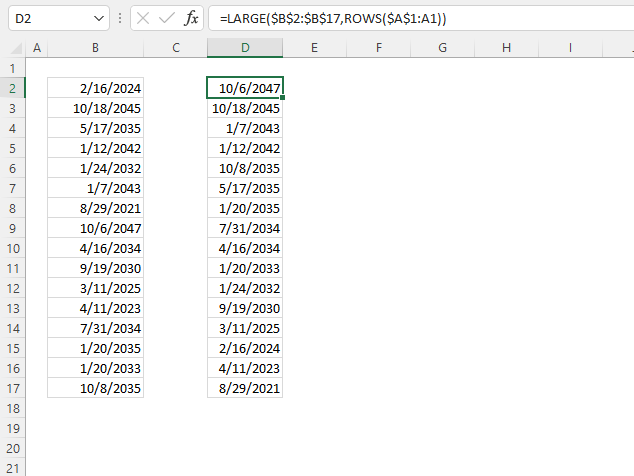Formula in cell D2:

=LARGE(\$B\$2:\$B\$17, ROWS(\$A\$1:A1))

### Explaining formula in cell D2

#### Step 1 - Create a sequence of values from 1 to n

The ROWS function returns a number based on the number of rows in a cell reference.

ROWS(ref)

ROWS(\$A\$1:A1)

returns 1.

Note that \$A\$1:A1 is a growing cell reference meaning it expands when you copy the cell and paste it to cells below.

#### Step 2 - Extract k-th largest serial number

The LARGE function calculates the k-th largest number.

LARGE(arrayk)

LARGE(\$B\$2:\$B\$17, ROWS(\$A\$1:A1))

becomes

LARGE(\$B\$2:\$B\$17, 1)

becomes

LARGE({45338; 53253; 49446; 51878; 48237; 52238; 44437; 53971; 49050; 47745; 45727; 45027; 49156; 49329; 48599; 49590}, 1)

and returns 53971 (10/6/2047) in cell D2.

Note, use the SMALL function if you want to sort the dates from oldest to newest.

## 9. Date function add days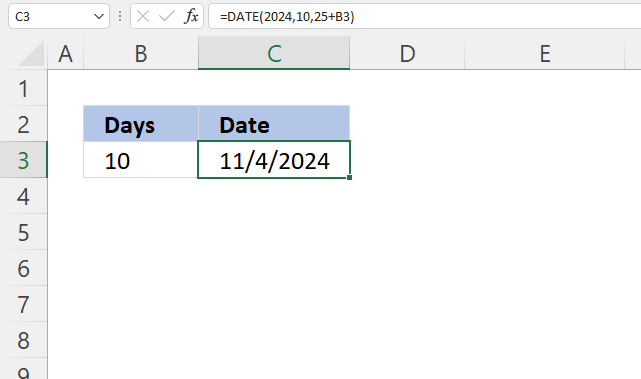The formula in cell C3 adds 10/25/2024 with 10 days specified in cell B3.

Formula in cell C3:

=DATE(2024,10,25+B3)

### Explaining formula in cell C3

#### Step 1 - Add dates

The plus sign lets you add numbers in Excel formulas.

25+B3

becomes

25 + 10 equals 35.

#### Step 2 - Calculate serial number representing an Excel date

The DATE function calculates a serial number that represents a specific date based on three arguments. Year, month, and day.

DATE(year, month, day)

DATE(2024,10,25+B3)

becomes

DATE(2024,10,35)

and returns 45600 formatted as 11/4/2024 in cell C3.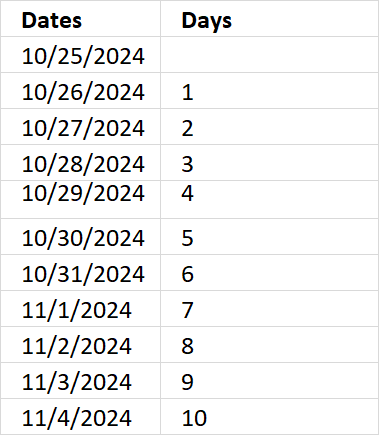## 10. Date function subtract days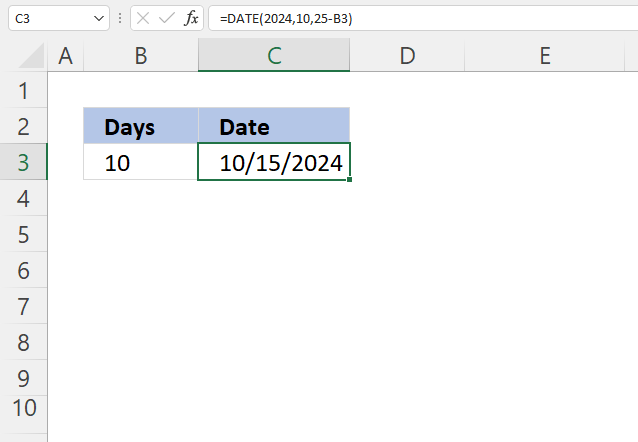The formula in cell C3 subtracts 10/25/2024 with 10 days specified in cell B3.

Formula in cell C3:

=DATE(2024,10,25-B3)

### Explaining formula in cell C3

#### Step 1 - Subtract dates

The minus sign lets you subtract numbers in Excel formulas.

25-B3

becomes

25 - 10 equals 15.

#### Step 2 - Calculate serial number representing an Excel date

The DATE function calculates a serial number that represents a specific date based on three arguments. Year, month, and day.

DATE(year, month, day)

DATE(2024,10,25-B3)

becomes

DATE(2024,10,15)

and returns 45580 formatted as 11/4/2024 in cell C3.

## 11. Date function add months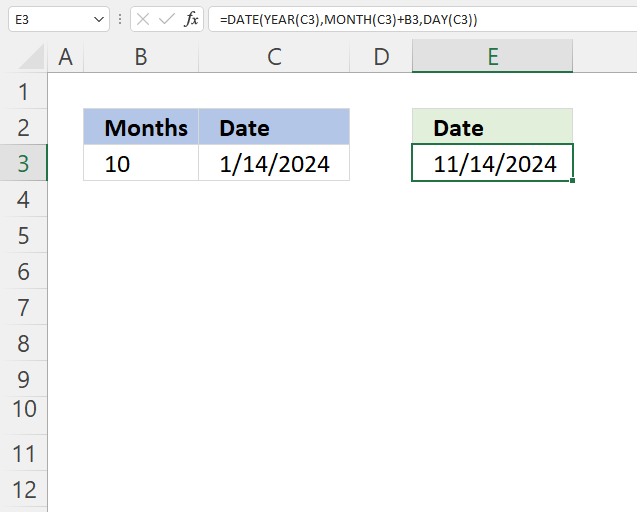The formula in cell E3 adds months based on the number in cell B3 to a date specified in cell C3.

Formula in cell C3:

=DATE(YEAR(C3), MONTH(C3)+B3, DAY(C3))

### Explaining formula in cell C3

#### Step 1 - Calculate year

The YEAR function returns a number representing the year based on an Excel date (serial number).

YEAR(serial_number)

YEAR(C3)

becomes

YEAR(1/14/2024)

becomes

YEAR(45305)

and returns 2024.

#### Step 2 - Calculate month

The MONTH function returns a number representing a month. 1 - January, ..., 12 - December.

MONTH(serial_number)

MONTH(C3)+B3

becomes

MONTH(45305)+10

becomes

1+10 equals 11.

#### Step 3 - Calculate day

The DAY function returns a number representing the day from an Excel date (serial number).

DAY(C3)

becomes

DAY(45305)

and returns 14.

#### Step 4 - Calculate date

The DATE function calculates a serial number that represents a specific date based on three arguments. Year, month, and day.

DATE(year, month, day)

DATE(YEAR(C3), MONTH(C3)+B3, DAY(C3))

becomes

DATE(2014, 11, 14)

and returns 45610 (11/14/2024).

## 12. Last day of a given month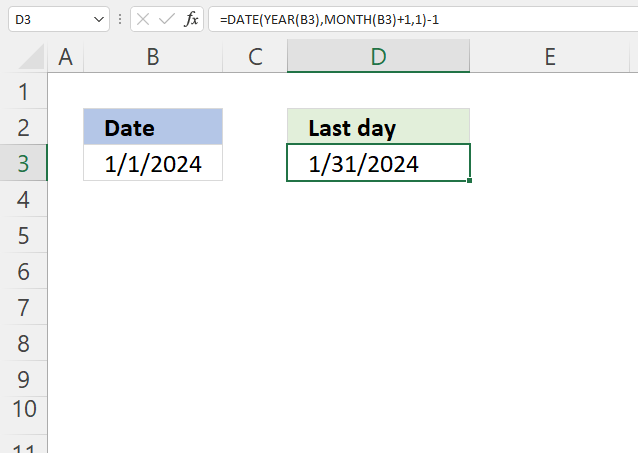The formula in cell D3 calculates the last day for a given month based on a date in cell B3. The date is 1/1/2024, the last day in January 2024 is 31.

The way this works is that the formula adds one month to the date in cell B3 then subtracts with 1 to get the last date of the previous month.

Formula in cell C3:

=DATE(YEAR(B3),MONTH(B3)+1,1)-1

### Explaining formula in cell C3

#### Step 1 - Calculate year

The YEAR function returns a number representing the year based on an Excel date (serial number).

YEAR(serial_number)

YEAR(B3)

becomes

YEAR(1/1/2024)

becomes

YEAR(45292)

and returns 2024.

#### Step 2 - Calculate month

The MONTH function returns a number representing a month. 1 - January, ..., 12 - December.

MONTH(serial_number)

MONTH(B3)+1

becomes

MONTH(45292)+1

becomes

1+1 equals 2.

#### Step 3 - Calculate day

The DAY function returns a number representing the day from an Excel date (serial number).

DAY(B3)

becomes

DAY(45292)

and returns 1.

#### Step 4 - Calculate the date

The DATE function calculates a serial number that represents a specific date based on three arguments. Year, month, and day.

DATE(year, month, day)

DATE(YEAR(B3),MONTH(B3)+1,1)-1

becomes

DATE(2014, 2, 1)-1

becomes

45323-1

and returns 45322 (1/31/2024).